ANALYZE menu File Edit Analyze View Script Window Special

GENERAL NOTES ON ANALYSIS PROCEDURES:

•    DATA BLOCKS:     If no data block is selected, only the RUNNING AVERAGE, PAIRS DIFFERENCES, and FFT (Fast Fourier Transform) analyses are available (and except for FFT, only in Active Screen mode).  For all other analyses a data block must be selected.

 To select a data block: Use the normal Macintosh click-hold-and-drag method, with the cursor within the plot area.  Move the cursor to the beginning point on the plot area and click once, then repeat this procedure for the end point (which may be on either side of the beginning point).  Alternately, blocks of predefined duration can be selected with single clicks (BLOCK WIDTH option, below).  Blocks can include multiple screens.  In ACTIVE SCREEN mode, mark the start of the block, then shift screens as necessary until the end of the block can be marked.

•   Once you have performed an analysis operation and want to select a new data block, click the 'Exit' or 'Cancel' button in the RESULTS window (or that window's close button).  The cursor will become a crosshair when within the plot area.This example of a block window shows the upper and lower limits of the data within the block.  This particular block (being analyzed with the Integrate option) also shows:

• left and right limits (indicated by the shaded areas)
• a proportional baseline between the start and end points (left and right limits)
• the channel label (at bottom left)

MORE BLOCK HANDLING AND ANALYSIS TOOLS:

•   When activated, the AUTOREPEAT option will repeat the most recently used analysis whenever you select a new data block.  This simplifies doing the same analysis on a series of different blocks, but it can be annoying if you wish to perform different analyses.  If autorepeat is on and you want to do something else, pick the new operation from the ANALYZE menu or from the 'toolbar' buttons at the bottom of the plot area.

•   At the bottom of most ANALYZE operation windows, the 'Print Window Image' button will send a copy of the window content to the printer or an image file (pdf, png, etc):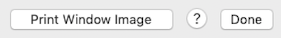•   Scaling factors may be applied to results for several ANALYZE operations with the 'scale' button (see below).

•    The 'Store' button in many of the analysis mode windows allows you to directly transfer the current results mean for use as a scaling factor.  When you click the 'Store' button, the scaling factors window appears.  Click on any channel's ' * ' or ' ÷ ' button, and the current mean will appear.

•    The 'help' button opens the LabAnalyst help window to the section for the ongoing analysis operation -- i.e., if you click 'help' from BASIC STATS, the help window opens to the basic stats instructions.

•    If the analysis operation you are using offers channel selection buttons, you can see the channel labels if you 'float' the cursor over the channel buttons*.   In addition, if you select the 'Show Channel Labels Windows' option in Preferences (LabAnalyst menu), a window will open near the bottom of the screen, showing a list of all the channels in the file.   Note that this window may not appear until you change channels or redraw the plot window.

* If you want to adjust the font size or the time delay for the 'Tool Tips' windows that show channel labels, and you feel comfortable entering text commands directly into MacOS, activate Terminal (Utilities folder, in Applications).   Then:

• To change font size to 14 points (or any value you like), type this (followed by RETURN):
defaults write -g NSToolTipsFontSize -int 14

• To change the activation delay to 20 ms (or any value you like), type this (followed by RETURN):
defaults write -g NSInitialToolTipDelay -int 20
• PRINTING RESULTS (text output):

Many analysis operations (and some other data manipulations) let you save results to a text file.  You can usually chose between two formats:•   Comma-separated variables (.csv).   This is a standard format for text files and is easily opened and read by many editing programs.   If you have Microsoft Excel (or Office) on your computer, these files generally show as this icon or something similar, depending on what software you have installed.   If these files are double-clicked, Excel will open them automatically.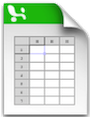•   Tab-separated text (ASCII) variables, which are saved with a suffix (.xls) that should make them open in Microsoft Excel if they are double-clicked or dragged.   NOTE: these are NOT in standard .xls format, which is a proprietary binary format that isn't created by LabAnalyst.   When Excel tries to open one of these files, it may give you a warning that the format is incorrect and ask if you want to load it anyway.  It should be fine to do so -- Excel quickly figures out that it's a text file and opens it correctly.

If no data block is selected you can perform the following two operations (as well as Fast Fourier transforms):

•   RUNNING AVERAGE...5  Lets you click on a series of SINGLE points while LabAnalyst keeps track of the cumulative mean, standard deviation (SD), coefficient of variation (CV), and standard error (SE).  The program also shows the summed total of all selected points, and the range (minimum and maximum values).

To select points for analysis, move the cursor to the point of interest in the plot area and click once.  Then move to the next point and repeat.

• You can eliminate a single mistake (the last point indicated with a mouse click) with the delete key.
• To exit the routine, move the cursor to the RESULTS window and click the mouse once (the cursor can be anywhere in the result window).

•    PAIRS DIFFERENCE...6  Calculates the cumulative mean, SD, and SE of a series of PAIRS of points.  You select point pairs with mouse clicks (an example might be the peaks and valleys of a ventilation trace).  LabAnalyst measures the absolute difference between the points in the pair.  It also calculates the cumulative mean, SD, and SE of the time intervals between points.  As for RUNNING AVERAGE, the delete key gets rid of the last pair of points selected.NOTE: this operation DOES NOT highlight the results window title when active, as can be seen in the example above.

If a data block HAS been selected, you can perform the following operations: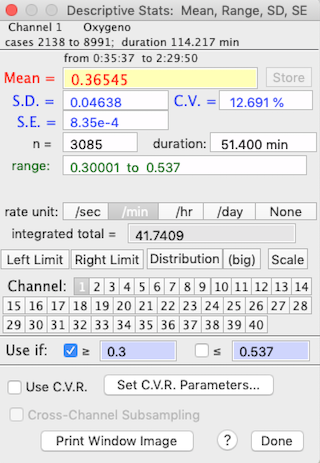•    BASIC STATSA; 'b'  Computes the mean, range, standard deviation (SD), standard error (SE), and coefficient of variation (CV) of the block (it also shows the time duration and the data range).   For convenience, this can be accessed from the keyboard by typing the ‘b’ character.

To get the integrated total for a rate function, such as oxygen consumption, make sure the correct rate unit is selected (i.e., 'per min' if the units are ml/min or 'per hour' if the units are ml/hour).  A more sophisticated integration mode is available in the INTEGRATE BLOCK option.

Use the 'limits' buttons to restrict analysis to a subsection of the block.  Click the right or left limit button, then use the cursor to select the limits in the block window.

See the notes on MINIMUM, MAXIMUM, LEVEL below for information on the 'C.V.R.' buttons.

The 'scale' button toggles scaling of the results (see SCALE RESULTS, below).  The 'Store' button in this and other analysis mode windows allows you to directly transfer the current mean for use as a scaling factor.  When you click 'Store', the scaling factors window appears.  Click on any channel's "*" or "÷" button, and the current mean will appear in the first edit field (the multiplication or division factor) for that channel.

The 'select,' '≥' and '≤' buttons at the bottom of the window let you get basic statistics for a subset of the block, within the defined numeric limits.   Note that there must be at least 3 data points within the subset limits, or the program will issue a warning buzz, turn off the '≥' and '≤' buttons, and default to the previous basic statistics results.If you restrict analysis to a subset of data, or use cross-channel subsets, a warning window will appear for analyses that 'pay attention' to subset settings.   This example shows cross-channel selection is being used, and within the channel being analyzed, data with values less than 0.5 or greater than 21.12 are ignored.

The 'distribution' button produces a small histogram (bar graph) of frequency distribution in a small moveable window. You can use the '(big)' button to produce a much larger and more detailed histogram, with control over the number of bins and bin width (see below). If the file has more than one channel, you can click on buttons for different channels and get those means (you can also use the keyboard to select channels). With an option in the PREFERENCES menu, you can have different histogram windows for each channel, or use the same small window for all channels.•    MINIMUM VALUE...M
•    MAXIMUM VALUE...U
•    MOST LEVEL...9
•    MOST VARIABLE...

Allow you to enter an interval (in seconds) and LabAnalyst will search within the selected block for either the highest or lowest continuous average over that interval, or the most level or most variable region within the block.   In this example, the scan interval (86,400 seconds) is an entire day.

NOTE: scanning large data blocks, especially for large scan intervals, can be time-consuming.   The program will post a warning message when it thinks calculation times will be lengthy.Activating the 'reuse this interval' button in the interval selection window will bypass the interval selection routine during subsequent uses of these analyses (such as when using the AUTOREPEAT option for new blocks).

You can restrict the analysis to specific ranges of data using the 'Exclude if' options.   In the example below, data with values less than 0.8 or greater than 2.3 are ignored during calculations.

For the MOST LEVEL option, the 'most level' region is the interval in which the sum of absolute differences from the interval mean (i.e., the sum of Xi - meanX, or point-to-point variance) is lowest.  Note that this is not necessarily the interval with the lowest slope, although this usually turns out to be the case.

For the MOST VARIABLE option, you can select to search either for the region with maximum overall slope or the region with maximum point-to-point variance.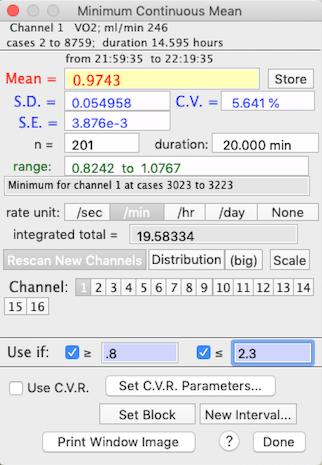When your interval selection is complete, click the 'interval OK' button and the program will find the appropriate interval and display the results in the next window, shown below.

After calculations, the maximal, minimal, or most level area is shown as a color-inverted rectangle on the block window.

As for BASIC STATS, you can switch to other channels, but in the default mode the interval boundaries remain constant -- i.e., the same beginning and ending points as on the initially scanned channel are used for other channels.  This sounds confusing but it allows you to scan for the period of, say, lowest VO2 and then get the temperature, CO2, etc. for that specific period.

Alternately, you can activate the 'rescan new channels' button to force a re-scan of each new channel selected.

Two other considerations:

• Rate units, distribution plots, storing data, and scaling are as described for BASIC STATS
• The 'Set block' button turns the sub-block (minimum, maximum, etc.) into the selected block for use in other analyses.If you use cross-channel subsets or restrict analyses to a specific data range, a warning window will appear for analyses that 'pay attention' to subset settings.  This example shows cross-channel selection is being used, and within the channel being analyzed, data with values less than 0.5 or greater than 21.12 are ignored.

When using the BASIC STATS..., MINIMUM VALUE...  and MAXIMUM VALUE... functions, two buttons, labeled 'Use C.V.R.' and 'Set C.V.R. Parameters...' are available. This stands for Cinstant Vplume Respirometry, and itopens a window that lets you set up the variables needed to compute O2 or CO2 exchange in a closed system.   In constant volume respirometry (or 'closed system' respirometry), the organism is placed in a sealed chamber, and over time its respiration changes the gas concentrations in the chamber.   You measure rates of gas exchange by determining gas concentrations (O2 and/or CO2 ) at the start and end of a period of measurement, and then using the cumulative difference in concentrations and the elapsed time to compute the average rate of change.

The most straightforward way to handle constant volume calculations with LabHelper and LabAnalyst is as follows:

• First, collect a sample or samples of 'initial' (unbreathed) and 'final' gas from the animal chamber(s) and inject them through a gas analyzer while continually recording the concentration (in %) with LabHelper.   If you have multiple samples, between injections of sample gas, flush the analyzer with reference gas (or fluid). You should get a data file with a series of 'peaks', one for each injection of 'final' gas (or fluid).

• Next, in LabAnalyst, use the baseline function to set the 'initial' values at zero.   The 'final' values now show the % change during the measurement period.  These peaks are what you analyze with the C.V.R. option.   For each peak, find the maximum deflection from baseline with the MAXUMUM VALUE option, set the C.V.R. parameters, and then switch on Use C.V.R..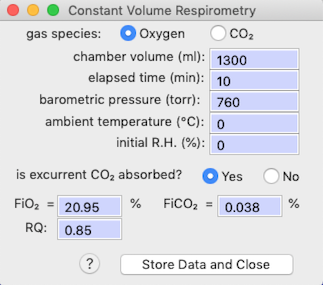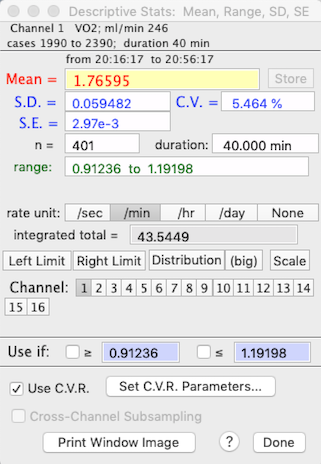Note that this option assumes that the data being analyzed are in units of % gas concentration and that baseline has already been corrected.   Click the 'Set C.V.R. Parameters...' button and, in the window that opens, specify gas type, chamber volume, elapsed time, chamber temperature, barometric pressure, initial relative humidity in the chamber (if the gas contains water vapor), the initial concentrations of O2 and CO2 (FiO2 and FiCO2), and the respiratory exchange ratio (RQ).  You also need to specify whether or not CO2 is absorbed prior to oxygen analysis ('is excurrent CO2 absorbed?' button).  When done, click the 'Store Data and Close' button.

When C.V.R. data... is activated, the results window (example on the right) shows gas exchange rates in units of ml/min -- but note that only the mean value is computed as gas exchange (the SD, SE, etc.  are shown in their original units).  To switch off the C.V.R.  calculations, click the 'C.V.R.  data...' button.   Note that this is a “quick and dirty” CVR estimate; a more versatile CVR calculator is in the SPECIAL menu.

You can avoid using interpolated data in these operations if you select the 'Avoid interpolated data' option in the ANALYSIS UTILITIES submenu (bottom of the ANALYSIS menu).•   INTEGRATE BLOCK...         Finds the area under the curve within the defined block.

There is a choice of time units (seconds, minutes, hours, days, none) and baseline values.  The baseline for integration can be set at zero, the initial value of the block, the final value of the block, a user-specified value, or a proportional linear correction between initial and final block values.  Because of the different baseline options, this operation is considerably more versatile than the integration feature including in BASIC STATS, MINIMUM, MAXIMUM, and LEVEL.

In this example, the block is integrated using the zero baseline option with the time unit set as minutes.  Note that the start, end, and proportional options apply to the block defined with the left and right limit functions.

Keep in mind that you need to pick the time unit that matches the rate unit used in the channel being analyzed, or the results will not be valid.  If you are using a rate unit for which a matching time unit is not available, you will need to adjust the results manually.  For example, if your data are in units of Kilojoules/day, you will need to divide the results by the factorial difference between days and whatever unit you select.  To continue with this example, if you set the units to 'hours' and your data are in KJ/day, you must divide the results by 24 (since there are 24 hours per day).  Similarly, if you set the units to 'minutes', you would need to divide by the number of minutes per day (1440).  This can be done conveniently using the 'Store' and 'Scale' buttons.If you select the "∑ plot" option (button in the bottom row), the computer generates a plot of the integrated values over time.  This plot will change whenever a new channel, right or left limit, or baseline option is selected.

• Use the '+', '-', and 'clear' buttons to add or subtract successive integral measurements.
• Use the 'limits', 'store', and 'scale' buttons as described for BASIC STATS.
• This operation prints to a tabular file but the output format isn't 100% compatible since there are no SDs generated during integration.

LabAnalyst contains three methods of analyzing the cyclical or waveform structure of a data set.  These are the WAVEFORM, TIME SERIES, and FFT (fast Fourier transform) operations.  All have different approaches, and the best one to use depends largely on the data set in question.

•   WAVEFORM...W         Uses simple algorithms to calculate wave frequency and mean peak height.  Basically, the program looks for successive 'peaks' and 'valleys' in the data.  A peak (the 'crest' of a waveform) is defined as a series of 5 points with the middle point having the highest value, and with values declining in the two points on either side.  The inverse is true for a valley (the 'trough' of a waveform).  LabAnalyst marks the peaks and valleys it finds with dotted (valleys) or dashed (peaks) lines in the block window (see below).In the default mode the program will use all the data within the block.  Alternately, 'filtering' is possible through cursor selection of minimum peak and valley values ('triggers') in the block window.  Click the 'use cursor' button and move the cursor to the block window.  A horizontal line will track the cursor's movement and a readout in the Results window will show the height of the cursor.  Click once to select a peak (done first) or valley trigger.  After selection, peak triggers are shown as pink lines, and valley triggers are green lines.  The post-peak trigger value (default zero) is the number of cases the program 'skips' after finding a peak.  This option can be useful when analyzing noisy files.A typical results window is shown at right, above.  Note that in this example the number of periods and amplitudes are not correctly matched (i.e., the number of amplitudes does not equal the number of cycles +1, indicating that some peaks were not associated with definable valleys, so the frequency or amplitude may be incorrect).   In that condition, the program beeps and prints a warning, as shown.   Warnings are also displayed if no periodicity is found, or if no amplitudes are found.  The mean values for both peaks and valleys are also shown.

NOTE: The waveform algorithms are easily confused by noise (because of the way peaks and valleys are defined).  If you are only interested in frequency, it is reasonably safe to reduce noise by smoothing data prior to analysis.  However, smoothing reduces peak amplitudes (in some cases very dramatically), so it must be used with caution if you need peak height data.  Smoothing is least damaging if peaks are 'rounded' and contain many more points than the smoothing interval.  If necessary, use PAIRS DIFFERENCE to obtain peak heights, then obtain frequency data after smoothing.The 'wave shape data' button opens a window with statistical information on the waveform's rise and decay times.

Rise time (the elapsed time from a valley to a subsequent peak) is shown in blue; decay time (the elapsed time from a peak to a subsequent valley) is shown in red.  The small triangles indicate the means while the bars show the distributions.

If the data contain more than 10 peaks, additional analyses are available from the Peak and interval histograms button, which produces histograms of peak height (shown as the absolute value), wave amplitude (valley to peak) and interpeak interval (essentially the wavelength calculated on a peak-to-peak basis), selected with a pop-up menu.  Another pop-up menu lets you select the number of bars in the histogram.  An example is shown below.

The save data button stores a text file of the histogram values, the print graph button sends the data to a printer, and the square-root Y button shows the count as a square-root, which better shows bars with low counts.•   Time seriesThis submenu has two items:

•   BASIC TIME SERIES...      This option displays time series data in graphical form.  Time series analysis examines data for many kinds of temporal relationships -- basically, does sample value at a particular time ( T ) predict sample value at some later time ( T + z)?   A graphical display is useful for determining if there are periodicities within the data, and (if periodicities exist) if the waveforms are symmetrical.  When this option is selected, LabAnalyst opens a window containing edit fields for the Start Lag (the initial time increment between samples) and the Time Step (the time increment added for each successive plot).  Defaults are a start lag of 0 and a time step of 1 sample.  Edit as desired.  There are two plotting options:

Use the 'Plot T versus T + step' button to generate 18 scatterplots.  For each, the sample value (X - coordinate) is plotted against the sample value at a fixed time increment in the future (Y -coordinate):

•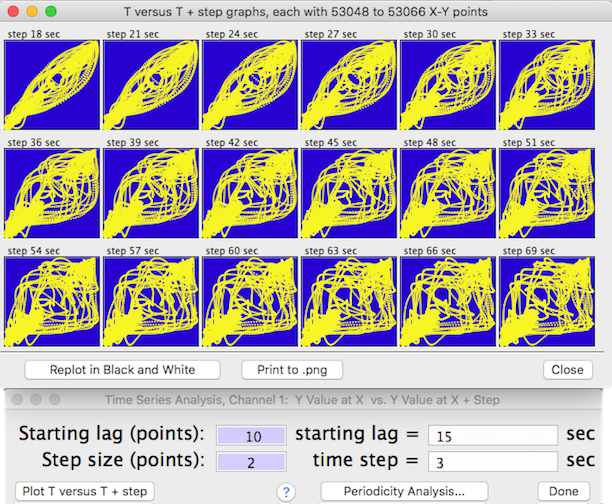In each plot the time increment is equal to the start lag plus the sum of the cumulative time steps (i.e., start lag + time step X plot number).  The increment value is shown at the top of each plot.  If there is no temporal predictability, the point distribution will be random.  However, if temporal patterns exist, the distribution of points will be non-random, and the shape of the distribution will indicate the degree of symmetry and the scatter will indicate the degree of randomness or 'noise'.  You can replot with different start lags and time step increments.  Click the 'Print to .png' button to make a file copy of the plots.   You can replot the window in black and white before saving it to .png.

• Use the 'Periodicity Analysis… ' button to generate a summarized periodicity test for 50, 100, 200, or more stepped time intervals (NOTE: the interval selection buttons are only available if there are sufficient points within the block).   For 200 or fewer steps, results are shown as a bar graph; for more steps a line plot is drawn. This is the setup screen for generating a periodicity test:Output from a typical periodicity test looks like this:This example shows a 750-step plot.  Correlations with negative slopes are plotted below the zero line.    The bar heights are a relative index of how value at time ( T ) predicts value at time ( T + time step).   The tallest bar (red on color screens) is the interval with highest predictability.  Click the 'Show Other Peaks Where...' button to display only those time correlations with r2 higher than a specified value.   Click the 'Copy to PDF' button (or 'Copy to PNG' depending on which text file format was selected in Preferences) to make a copy of the graph.   Click the 'Done' button or the close box to exit.

•    STEPPED SAMPLING...     This routine computes, plots, and saves sequentially sampled data at user-set intervals; among other uses, this helps determine the degree to which data sampled at one time are correlated to data sampled at different times (i.e., autocorrelation).  For example, if you wanted to compute a relationship between (say) voluntary running speed and metabolism from a file containing many different running speeds, you would need to obtain your measurements at large enough intervals so that there was no autocorrelation between measurements.  The intuitive expectation is that successive measurements obtained at very short intervals will closely resemble each other, but eventually become independent as the interval between measurements increases.  The goal would be to use an inter-measurement interval large enough to be sure that autocorrelation ('pseudoreplication') is minimal.

The iterative sampling process starts at an initial point (a particular sample) in the file, then steps forwards and backwards by user-defined intervals (the 'skip' interval) and takes the mean, range, and SD of blocks of data of user-set duration, symbolized as:

-------||||||||-------|||||||--------|||||||-------||||||||-------

where ----- = skipped samples, ||||| = blocks, and | is the initial point

This process is repeated until user-defined limits are reached, or the start of end of the data are reached.  The initial point can be a user-set sample number or the central point in a data block (for example, you could search for the highest point in a data file, use the 'set block' option, and then use the resulting block as the initial point).  If a block is selected, the start and end points are set to the beginning and ending points of the block.  The default value is 1/2 of the total number of samples. The control window looks like this:When ready, click either the "delta-t step plot" button or select a new channel to display results, as in the following example:Results are plotted on-screen (blue for measurements later than the initial point, red for measurements earlier than the initial point, and gray for the mean value for both + and - values at a given interval).

The 'scatterplot' button shows an X-Y scatterplot of step-sampled data from any two of the available channels (it's only available if there are 2 or more channels in the file).

You can use the channel buttons to select up to 10 channels that can be analyzed and stored in a tab-delineated (Excel compatable) or .cvs file with the "save as ASCII" button.  Note that you can select more than 10 channels, but only results from the first 10 will be stored.  If the file contains interpolated data as indicated with the standard interpolation markers "»" and "«", any stored values that were computed from interpolated data will be marked in the Excel file.

•    FFT...     Uses a Fast Fourier Transform (FFT) to calculate the frequency structure of cyclic data.  Theoretically, any waveform can be decomposed into a set of simple sine waves of different frequencies and phases.  The FFT algorithm finds these fundamental frequencies and displays them as peaks in the plot area.Complex 'summed' frequency data occur frequently in biology (and in other areas of science).  For example, you might want to use an impedance converter to measure the heart rate in a small mammal, bird, or lizard.  Unfortunately, in addition to heart rate, you will also pick up signals produced by breathing movements.  Therefore the instrument output will contain a confusing summation of the combined effects of breathing and heart rate.  It may also contain 'noise' from random or irregular events (such as muscle movement from minor postural adjustments).

The messy-looking data shown at right are an example of such a waveform.  Although it is obviously complex, a visual inspection suggests that it does contain some regularity.  However, this periodicity is not readily studied with either the WAVEFORM or TIME SERIES operations.  Fortunately, the FFT procedure can help find the important underlying components of this complex wave.  In many cases it can detect basic cycles in a data set even if they are visually 'buried' by random noise.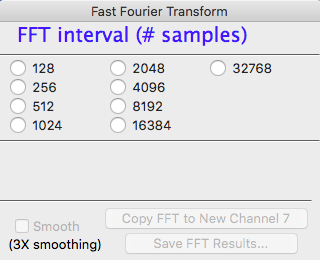The first step in the FFT procedure is selection of the size of the block of data to be analyzed.  The FFT algorithm requires that the block size be a power of two; depending on the size of your data set you can select any block size from 128 samples up to a maximum of 262,144 (256K) samples (in this example, the total number of samples in the file was about 700; accordingly, the largest possible block for FFT analysis is 32,568 samples).

After you select a block size, the program will show the block duration and then prompt you to go to the plot window and select the block to be analyzed.  Do this by moving the cursor into the plot area, where it will outline a block of the size you selected.  Fit the cursor block over the subset of data you wish to analyze and click the mouse once.  This will select the desired FFT block.

Once the block is chosen, you can proceed transform it (Do FFT button), select another block size ( ‘∆ interval’ ), or exit.  You may choose whether or not the results are smoothed; they are always shown initially as 'raw' FFT scores.After completing the FFT, the waveform's fundamental frequencies are shown graphically in the plot area.  An example from the waveform shown in the first image above is shown at right.   Note that the complex-appearing waveform can be described as the combination of three fundamental frequencies, shown in the plot area as three sharp peaks.   You can examine the details of this structure by moving the cursor over the plot; the fundamental frequencies that have been 'decomposed' from the original signal, and their amplitudes (which are arbitrary but 'proportional' to other peaks and hence are useful for comparing among them), are shown numerically as peaks in the results window.   An example of the FFT results window is shown below.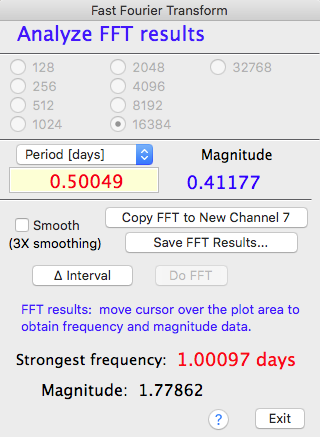In this example, the analysis was performed on 16,384 points, and the strongest (most fundamental) underlying frequency in the waveform was almost exactly one day (this is unsurprising, since the data are of environmental temperature variation over several months).  The cursor is over one of the peaks, which corresponds to a period of 0.5 days and a magnitude (useful for comparisons among peaks) of 1.78 (magnitude data are displayed in the plot window data bar).  You have a choice of output units (frequency in Hz, kHz, etc.; period in sec, min, etc.).   The software will attempt to use the most reasonable units.

After the transform is complete, you can expand or shrink the plot window display using the spacebar or return key (similar to the regular 'entire file' versus 'one screenful' display modes modes), or smooth (or unsmooth) the data.

FFT results are stored in channel zero (not normally used by LabAnalyst); use the copy button to move them to a 'regular' data channel if you want to save them to disk (copying is only possible if the number of 'regular' channels is <40).  Alternately, you can use the 'save FFT…' button to produce an Excel-compatible or .cvs spreadsheet containing the frequency and magnitude data (saved in whatever frequency or period you selected with the popup menu) and amplitudes.

Note that if you click the exit button, you are transferred back to the original data channel.  You cannot get back to the FFT results in channel zero except by re-running the FFT procedure.

•   SLOPE vs TIME...1 (numeral 1)  Calculates rates of change over time within a data block.  Seconds, minutes, hours, or days may be selected as the time units.  Either linear (least squares) or semi-log regression (log Y vs. time) can be selected.  The semi-log option is not available if any Y values are negative.  When the calculations are complete, a regression line is superimposed on the block window, and the program computes slope, r-squared, and probabilities that slope = 1 or 0.  You can check the slope against other values using the 'test' button.

Two values of 'a' (the intercept) are given.  One is based on the time change calculated from the start of the file (t=zero), and the other is based on the time change within the block, using the assumption that time zero is the start of the block.You can use the 'predict values' option to calculate specific Y values as a function of time, or vice versa (this uses the intercept value calculated from the start of the block, not the start of the file).  At present, this does not 'back-convert' from log to linear, so if your equation uses log time and log Y, you will need to convert your inputs to their log equivalents, and do the reverse for predicted values.

You can switch channels (for new calculations) with the usual selection buttons on the bottom of the results window.  To change the time units, re-select the original channel.

You can also test the slope against any user-defined value with the 'test' button.  The 'Residuals…' button draws a plot of the residuals from the regression; results -- time, observed value, predicted value, and residual -- can be saved to an Excel-format text file.

• CAUTION:   plotting residuals from a large data block (> 10,000 cases) may cause the program to 'beachball' for a long time -- be patient!

• Note that in SLOPE and REGRESSION you cannot send results to disk or printer by hitting the 'p' key, as usual.  Instead, use the 'print' button (this button only appears if output has been selected from the FILE menu).
• This option DOES NOT print to a tabular file (the output format is incompatible).

•   REGRESS CHANNELS...2  This option performs basic regression analysis for any two channels (obviously, it is only available if the file has more than one channel):The initial step in the regression procedure is to chose the type of unit conversions.  Linear (least-squares), semi-log (log Y = a+b*X or Y = a+b*log X), and log-log (log Y = a + b*log X) regression models are available.  However, some of the conversions will not be available if the data range includes zero or negative values (since one can't take the log of a negative number).

Next, you select the two channels to regress, using the window shown at right.  The program won't let you attempt to regress a channel against itself, so you may have to do some fancy button-clicking to get your channels selected.After the 'selections OK' button is clicked, LabAnalyst performs the calculations and produces a scatterplot of the data points in the block window (x values versus y values), along with the regression line.

NOTE: regressing large data blocks can be time-consuming.   The program will post a warning message when it thinks calculation times will be lengthy.

The numerical results are the same as for the SLOPE vs TIME option described above -- except that only a single value of the intercept is shown.

You can test the slope against any user-defined value.  Enter the slope into the edit field and click the 'test' button (very low probability values are shown as "<.00001").

The 'residuals' button will produce a scatterplot of residuals from the regression.   'Select new channels' lets you set up a new regression of different variables.You can use the 'predict values' button to use the regression equation to predict X from a given Y, or vice versa:

• Note that in SLOPE and REGRESSION you cannot send results to disk or printer by hitting the 'p' key, as usual.  Instead, use the 'print' button (this button only appears if output has been selected from the FILE menu)
• This option DOES NOT print to a tabular file (the output format is incompatible).

•   FIND ASYMPTOTE...3  This is an application of first-order kinetics, in which data approach a stable plateau (asymptote) according to a rate constant (this means that the fractional rate of approach to the asymptote is constant over time).  Some examples include heating and cooling (the "Newtonian" model) and gas mixing and washout characteristics (and many other physical phenomena).

The program uses an iterative method to find the best-fit asymptote for a selected block of data, according to the simple model: Y = ln (asymptote - data).  You can chose any number of iterations between 3 and 50 (the default is 6).  Using a lot of iterations might increase the accuracy of the estimate (this doesn't always occur), but will also increase the analysis time.  In practice, you usually don't need to use more than 6 to 10 iterations for good accuracy.  Note that if you give it 'messy' data that do not conform reasonably well to first-order kinetics, the program may take a long time to produce an estimate (and that estimate may have fairly glaring errors).After completing the analysis, LabAnalyst shows the asymptote, the coefficient of determination or C.D. (an estimate of the precision of the fit of the data to the model, and hence the precision of the estimated asymptote), the rate constant (the fraction of the change between a starting value and the asymptote that is completed during 1 time unit), and the time to complete a fraction of the total change between a starting value and the asymptote (values from 1% to 99% are selectable from a pop-up menu).  You can use your choice of time units (seconds, minutes, hours, or days) for slopes and rate constants.

For relatively small block sizes, LabAnalyst also draws a goodness-of-fit plot that illustrates how closely the model matches the data for large blocks you can get this plot by clicking the 'Show Regression' button.  CAUTION:   for large files (e.g, > 1,000,000 points), plotting is a slow process.  As shown above at right, points are plotted as the log (base e) of the absolute difference between the model predictions and the data:

Y value = ln(abs(asymptote - data))

Individual points are shown only if the total number of points in the plot is less than 60.  In these examples, data are plotted in yellow on a red background and the best-fit regression line predicted by the model is shown in white.  You can select other color combinations in the 'Plot Styles' option in the VIEW menu.   After the goodness-of-fit plot is drawn, you can also show the residuals from the regression, as shown below (Caution: this is slower than drawing the regression itself):• The time needed for finding an asymptote increases with the size of the data block (as well as the number of iterations).  On a typical machine, a block containing several hundred thousand data points will be analyzed within a second or so. For large data blocks, the program will put up a notification that processing may be lengthy.   Note that plotting the data takes much longer than analyzing the model fit!

• You cannot send results to disk or printer by hitting the 'p' key, as usual.  Instead, use the 'print' button (this button is available only if output has been selected from the FILE menu).

• This option DOES NOT print to a tabular file (the output format is incompatible).

•    FIT POLYNOMIAL...4       In some cases, relationships between different variables are best expressed with a polynomial regression.  LabAnalyst lets you fit pairs of channels with polynomials of up to 9 degrees.  The model is:
Channel Y = (polynomial expression of Channel X).
After you select the X and Y channels (as described above for linear regression), the program computes and displays a default polynomial of 3 degrees.  Regression statistics (mean and SD of X and Y, residual Y variance, and r2) as well as the polynomial coefficients are shown.   You can then select other degrees using the pop-up menu.  In this example, a 6-degree polynomial was used to predict CO2 concentration from oxygen concentration; the resulting equation -- shown at the right of the window -- explains 99% of the variance in % CO2.After completing the analysis, several options are available.  The 'Pick New Channels' button lets you change the X and Y variables.  The 'Make New Chan From Polynomial' button (only available if the number of channels is less than 40) generates a new channel by applying the current polynomial equation to the data in the X channel -- in other words, it computes and saves a new Y value for every sample in the X channel.The 'Show All r^2' button helps you select the most appropriate degree for the polynomial equation.  The predictive value of a polynomial always increases as the degree increases.  However, the increase in accuracy usually plateaus, so it is reasonable to use the simplest equation consistent with good predictive power.  When the 'Show All r^2' button is clicked, the program computes the r2 value for all degrees between 1 and 9, and then shows a bar graph of the results.  [You can remove this plot by clicking the highlighted 'Ahow All r^2' button.]

In the example shown at right, there was little change in predictive power until the degree of the polynomial exceeded 3, and then little additional change until the degree reached 8 and 9 (you can select the color of bar graph plots in the Colors and lines option in the VIEW menu.)

• In some conditions (particularly if you are computing a high-degree polynomial when the magnitudes of the X and Y channels differ greatly), it is possible to exceed the numeric limits of the program.  Usually, the value of r2 is set to zero when this happens.
• You cannot send results to disk or printer by hitting the 'p' key, as usual.  Instead, use the 'Print' button (this button is available only if output has been selected from the FILE menu).
• This option DOES NOT print to a tabular file (the output format is incompatible).

•   TIME INTEGRATION7   This operation calculates the cumulative duration of data in the selected block that satisfy two Boolean criteria: greater than one user-selected value, and/or less than a second user-selected value.

An example time integration window is shown at right.  The default minimum and maximum values are the lower and upper limits (respectively) of the data range in the block, which includes 100% of the data and results in a single event in each category. You can set new limits (as shown here) in three ways:

• When the 'minimum' or 'maximum' buttons are clicked, the limits are reset to these values
• When the 'greater than' or 'less than' buttons are clicked, you can use the mouse to move cursor in the block window to graphically select the upper and lower limits.
• Finally, you can directly type in the limit values in the edit fields (hitting "return" will force the program to recalculate the results).
Be sure to select the time unit you want.  Clicking the 'Switch to Selective integration' button changes to that mode, using the current set points.
• You cannot send results to disk or printer by hitting the 'p' key, as usual.  Instead, use the 'print' button (this button only appears if output has been selected from the FILE menu)
• This option DOES NOT print to a tabular file (the output format is incompatible).

•    EVENT COUNTING8    This operation counts the number of 'events' in the selected block that satisfy two Boolean criteria: greater than one user-selected value, and less than a second user-selected value.  An 'event' occurs when the data cross one of the two Boolean 'boundaries'.  A 'negative' event is counted when the data drop below the lower limit, and a 'positive' event is counted when the data rise above the upper limit.   The default minimum and maximum values are the mean of the lower and upper limits of the data range in the block, which includes 100% of the data and results in a single event in each category.  An example of event counting is shown below.You can set new limits in three ways:
• When the 'minimum' or 'maximum' buttons are clicked, the limits are reset to these values
• When the 'greater than' or 'less than' buttons are clicked, you can use the mouse to move cursor in the block window to graphically select the upper and lower limits.
• Finally, you can directly type in the limit values in the edit fields (hitting "return" will force the program to recalculate the results).
• You can include all the events, or elect to analyze only events longer than a minimum duration.   Toggle these options with the 'count all events' button.

The right side of the window shows basic statistics for the durations of positive and negative events (mean, SD, range), and a histogram of these values.   Only complete events (including both beginnings and endings) are shown here.  You can elect to analyze only events longer than a minimum duration.   The 'show all events' button forces the basic event counters to show all events, not just those longer than the minimum duration.
The 'save details' button makes a text file (Excel format) containing the polarity (positive or negative), start time, duration, and basic statistics (mean, SD, minimum, maximum) for each identified event.  Some additional considerations include:

• You cannot send results to disk or printer by hitting the 'p' key, as usual.  Instead, use the 'print' button (this button only appears if output has been selected from the FILE menu)
• This option DOES NOT print to a tabular file (the output format is incompatible).•   SELECTIVE INTEGRATION     This operation integrates all data in the selected block that satisfy two Boolean criteria: greater than one user-selected value, and less than a second user-selected value.  The window shows the integrated value in absolute terms and as the fraction of the total integrated block.

An example of selective integration is shown at right.  The default minimum and maximum values are the lower and upper limits (respectively) of the data range in the block, which includes 100% of the data and results in a single event in each category.  You can set new limits (as shown here) in three ways:

• When the 'minimum' or 'maximum' buttons are clicked, the limits are reset to these values
• When the 'greater than' or 'less than' buttons are clicked, you can use the mouse to move cursor in the block window to graphically select the upper and lower limits.
• Finally, you can directly type in the limit values in the edit fields (hitting "return" will force the program to recalculate the results).
• You cannot send results to disk or printer by hitting the 'p' key, as usual.  Instead, use the 'print' button (this button only appears if output has been selected from the FILE menu)
• This option DOES NOT print to a tabular file (the output format is incompatible).
Additional considerations for TIME and SELECTIVE INTEGRATION and EVENT COUNTING:
• The 'distribution' button produces a histogram (bar graph) of frequency distribution in a small moveable window.  If the file has more than one channel, you can click on buttons for different channels and get those means (you can also use the keyboard to select channels).  With an option in the PREFERENCES menu, you can have different histogram windows for each channel, or use the same small window for all channels.
• You cannot send results to disk or printer by hitting the 'p' key, as usual.  Instead, use the 'print' button (this button only appears if output has been selected from the Output Menu).
• CROSS-CHANNEL SUBSET SELECTION... This window lets you perform some analysis function (average, integrate, etc.) on a subset of the data, based on the values in one or more other channels.  For example, you could calculate the average oxygen consumption in channel 4 only when running speed (channel 3) is greater than 0.5 meters/sec and temperature is less than 20 °C.
Choose your selection criteria according to three Boolean criteria: channel values greater than, less than, or equal to, a user-set index.  Click the appropriate button(s) in the channel(s) of your choice, and enter your selection indices.
• You can use multiple selection criteria in a single channel, as long as they aren't mutually exclusive (for example, using within-channel criteria of 'greater than 50 and less than 40' will eliminate all possible values).   You can also use multiple criteria in different channels.
• Analysis operations that permit cross-channel subset selection show a check button (at the bottom of the analysis window) for activation and deactivation of this feature.
• Edit fields that contain no visible numeric values are assumed to contain a value of zero.
• NOTE: To finalize your selection, you must click the 'set index' button.   When you do this, all of the data in the window are recorded, but only values with checked buttons will be used.

•   Analysis utilitiesThis submenu has four items:

•    AUTOREPEATR     Toggles the auto-repetition feature for analysis modes.  When active, autorepeat mode repeats the last analysis used whenever a new block is selected.  If you want to do something different, just pick the new analysis from the menu or from the buttons below the plot area.•   BLOCK WIDTH...         Allows automatic selection of blocks of user-defined duration with a single mouse click, instead of the normal method of clicking on both the start and end of the desired block (or clicking and dragging).  In this mode, the cursor is contained within a box that 'frames' the block duration in the plot area.  Position the cursor over the desired block and click once to select it.

The default automatic block size is equivalent to 13 samples or 12 sample intervals (e.g., 60 seconds with a 5-second sample interval).  Any other block size can be selected, as long as it contains more than 2 samples and less than 1/4 of the file duration.   In this example, a 5-minute block duration (300 seconds) has been selected.

Return to the standard two-click selection method with the 'Normal block selection' button.

•    SCALE RESULTS...      Opens a window for entry of scaling factors that can be selectively applied to the results of several analysis operations with the 'scale' button (in the RESULTS window).  These factors take the form:

final value = (result x B) +A or final value = (result ÷ B) +ADifferent A and B values can be applied to each channel, but you must enter the correct values in any channel you wish to scale (in other words, if you have scaling factors entered for channel 5, they apply only to channel 5 unless they are also entered in another channel of interest).

This example shows:

• addition of .74 to channel 1
• multiplication of channel 3 by 0.140479, and addition of .002 to the result.
• division of channel 5 by 2.
• no change to the other channels.

•    The 'Store' button in many of the analysis mode windows allows you to directly transfer the current results mean for use as a scaling factor.  When you click the Store button, the scaling factors window appears.  Click on any channel's "*" or "÷" button, and the current mean will appear in the first edit field (the multiplication or division factor) for that channel.

•    CROSS-CHANNEL SUBSET SELECTIONUse this option to restrict analysis according to specific ranges or values of data in another channel.   In the example shown here, data during the night are ignored (i.e., times between 18:00 and 06:00), as are data where temperature (Ta) is hotter than 65 or colder than 0.5.  It is not used in the example, but restricting to single values is possble (using the '=' option), but this is very restrictive and is probably best suited to boolean data (zero or one, for example).

If you use this option, make sure your restrictions leave SOME data available for analysis -- this is especially important if you are setting restrictions on more than one channel.If you use cross-channel subsets, a warning window will appear for analyses that 'pay attention' to subset settings.   This example shows cross-channel selection is being used, and within the channel being analyzed, data with values less than 0.5 or greater than 21.12 are ignored.

•    DON'T USE INTERPOLATED DATA       If this option is selected, the AVERAGE, MINIMUM VALUE, MAXIMUM VALUE, MOST LEVEL, MOST VARIABLE, SLOPE OVER TIME, and REGRESSION operations will not use interpolated data when scanning for desired values. Note that it does not matter which channel was interpolated: if this option is selected all data from any channel within interpolation boundaries will be rejected. Note that interpolation is determined from the standard interpolation markers: "»" indicates the start of interpolation and "«" indicates the end of interpolation. These are optionally set automatically in the Remove references and Interactive spike removal operations, or you can insert them manually from the 'markers' submenu in the VIEW menu.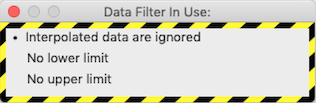If you have selected this option and an analysis operation encounters interpolated data within the regions selected for analysis, this warning window is shown:

You will also notice that the number of cases shown in the 'Results' window is less than shown in the 'Block' window. The difference is the number of interpolated data points that were ignored during analysis.

•    BLOCK SHIFT LEFT <<<      If there is room in the plot area, this will shift the block to the left (i.e., towards earlier events) by one blockwidth of data points.

•    BLOCK SHIFT RIGHT >>>     If there is room in the plot area, this will shift the block to the right (i.e., towards later events) by one blockwidth of data points.

•    BLOCK SHIFT RULES      This opens a small window that lets you select how the shift block operations work: with or without overlap of one case.  For example, if your block contains cases 200-400 and you shift it right with overlap, the new block will contain cases 400-600.   If you use the default non-overlap option, the new block will contain cases 401-601.